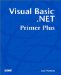# Chapter 9. Arithmetic and Assignment Operators

 You will learn the following in this chapter: What arithmetic operators are What an assignment operator is What a unary operator is What a binary operator is What operator precedence is How to change operator precedence This chapter explores the various assignment operators that are available in Visual Basic .NET. First, it discusses the operators that are used in arithmetic expressions. After that, it explains the assignment operators. You have used the assignment operator in numerous programs already, but as you'll learn in this chapter, there are some very useful variations that you need to know about.Visual Basic .NET Primer Plus
ISBN: 0672324857
EAN: 2147483647
Year: 2003
Pages: 238
Authors: Jack Purdum

Similar book on Amazon